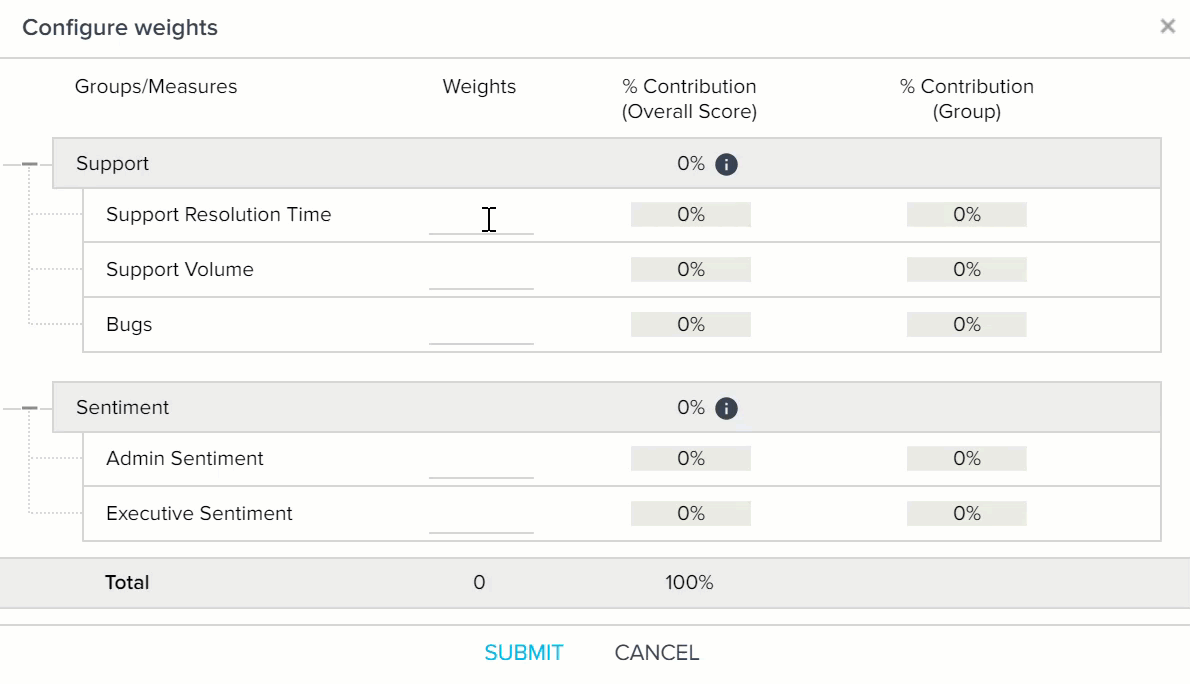Measure Weights in Scorecards

Gainsight NXT

This article supports Gainsight NXT, the next evolution of the Customer Success platform. If you are using Gainsight CS Salesforce Edition, you can find supporting documentation by visiting the home page, and selecting CS > Salesforce Edition.

This article explains how you can set measure weights in Scorecard. Gainsight recommends that you first read the Configuration section of the Create Scorecards article, so that you can understand where exactly measures are configured in Scorecard setup.

Assign Weights

A Scorecard is made up of Measures. Each measure has its own score and the collective combination of all the scores from all the measures determines the overall score for a Scorecard.

You can configure the way in which individual measure’s scores are combined to form the overall health score of a Scorecard, by applying Weights. You can assign a numerical value (weight) to all the Measures used in a Scorecard in the Weights column. The system auto converts the assigned numerical value into a Percentage value for every Measure and also for the Measures weight for the respective groups, and displays them in the % Contribution to Overall score and % Contribution (Group) columns respectively. The Percentage weight of every measure determines its weightage in the overall health Score. You can use any number (weight) from 0- 999999, as a measure weight. It is not required that the sum of all the weights are equal to 100. The sum of the weights can be any value. The system ensures that the sum of all Percentage values is equal to 100%, irrespective of the weights assigned by you. Currently, you can assign integer and floating point numerals as Weights.

Note: The % Contribution (Group) column is only displayed when you add Measure Groups to the Scorecard.You can either set the same weight for all of the measures, or different weights for each measure. If you feel that a particular measure should have a greater impact in the overall score, just assign the highest numerical value to that measure, so that its corresponding Percentage value is highest compared to other measures. Similarly, when you have multiple measures with varying levels of significance, assign weights accordingly such that the most significant measure gets the highest Percentage value, followed by the next most significant measure, whose Percentage value is lower only to the most significant measure, but higher than rest of the measures, and so on.

As depicted in the above diagram, you can use any numerical value in the Weights column. The system auto converts your numerical value into a Percentage value. The weight of a measure does not have any impact on the score of the measure. Weight of a measure only determines its weightage in the overall score.

You can also use floating point numbers as weights. Similar to integers, the sum of Weights can be any value; the system auto converts the floating point numbers into their Percentage equivalents. You can use floating point numbers up to two decimal points.You can also use a combination of integers and floating point numbers.You can also exclude one or more measures from the calculation of overall score, by not assigning any weight to it. If a measure does not have any weight assigned, it’s not considered in the calculation of the overall score. However, it still has its own health score.